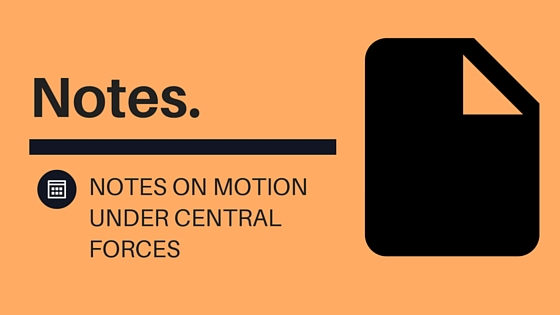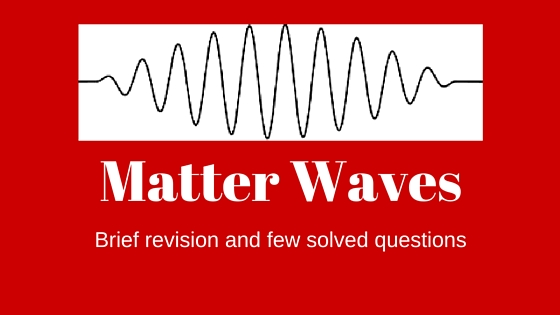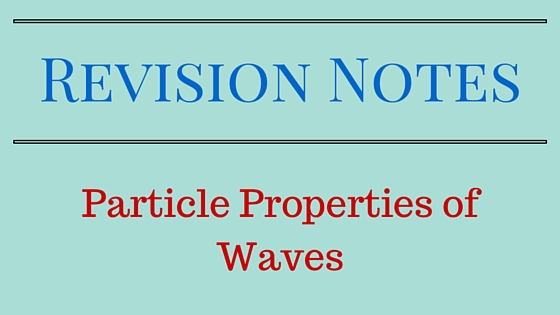# physicscatalyst## Motion Under Central Forces (part 2)

This article covers topics like Law of conservation of energy, Equation of motion and Form of motion under the effect of central forces.

## Law of conservation of energy

From equation 4 we consider $f(r) = – \frac{{du(r)}}{{dr}}$ then this shows that $f(r)$ is not only a central force but a conservative force. In this condition this equation## Motion Under Central Forces (part 1)

This article covers an introduction to central forces , equation of motion under central forces and

## Introduction

If the force $\mathop f\limits^ \to$ acting on a body has following characteristics then it is a central force (i) it depends on the distance between two particles (ii) it is always directed towards or away from a fixed point. Gravitational force is an example of central forces. Mathematically if we consider central point as origin## Matter Waves

This article is about matter waves and it contain brief introduction of the topic followed by few questions. Photoelectric effect and Compton scattering are evidences of the corpuscular nature of light. Interference , diffraction and polarisation reveal the wave nature of light. Louis de Brogli was the first one to suggest that matter may have …## Particle Properties of waves (Revision Notes)

This article is about the revision of Particle properties of wave. I’ll be doing detailed articles on each one of the sub-topics given in this article. Classical Mechanics do not put any restriction on values of a dynamical property of a particle, so small and large both values are permitted. Principle of superposition:- When two …

## Total energy of earth in its circular orbit around the sun

Question : Find out the total energy of earth in its circular orbit around the sun in terms of gravitational constant Answer: Let R be the total distance between the earth and the sun. If